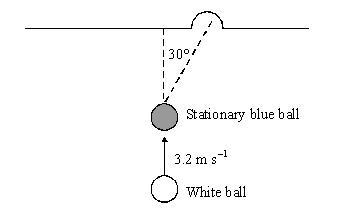# Conservation Of Momentum

Air

## Homework Statement

This diagram shows a white snooker ball moving with a velocity of $3.2 \ ms^{-1}$. The white ball is on a collision course with a stationary blue ball of identical mass of $0.173 \ kg$. The player is aiming to propel the blue ball into the pocket along the path shown.The player succeeds in propelling the blue ball along the path shown. The blue ball has a velocity of $2.77 \ ms^{-1}$ after the collision with the white ball. Calculate the velocity of the white ball after the collision.

## Homework Equations

$P=mv$ (I think)

## The Attempt at a Solution

As momentum is conserved, I would have momentum before equals momentum after hence...

$(0.173)(3.2) = (0.173)(v) + (0.173)(2.77) \implies v = 0.43 \ ms^{-1}$

But this is incorrect. Can someone explain how I would tackle this question and point out my mistake. Thanks in advance.(The correct answer is $v = 1.6 \ ms^{-1}$.)

Last edited: